# Algorithms Introduction

Algorithms as you must have read is simply “A step by step solution to solve a given problem.” So what do we mean when we say algorithms while talking in respect to computer problems ? So lets understand algorithms first with the help of a analogy.

We can view an algorithm as a machine or a factory. So what a machine or a factory does ? It takes a input or a set of inputs and performs a series of well defined procedures and functions to produce an output.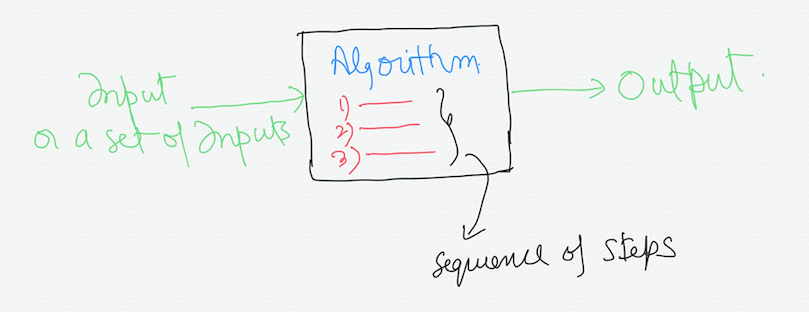This is just a vague description of algorithms. So let us take a more concrete example. One of the most important problem in computer science is of Sorting.

Sorting is a way to arrange a list of randomly arranged numbers, words, etc. into ascending(lowest to highest) or descending order(highest to lowest) order. In case of words, they can be arranged in albhabetically increasing(A->Z) or decreasing(Z->A) order. Now lets see the inputs and outputs of our Sorting algorithm machine.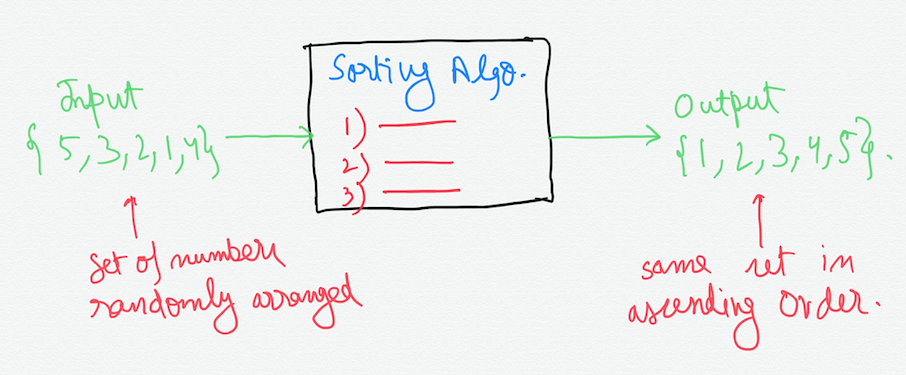So now we have got a problem in hand that of arranging the set of random numbers in ascending order. This sorting is easy enough for the human mind, but computer being a dumb machine has to be given a sequence of concrete steps to solve this problem or any problem for that matter.

We call this sequence of steps as algorithm. When these steps are written in a language(programming language) independent way, then it is called **pseudocode. **Also an algorithm may be correct or incorrect.

A correct algorithm is the one which always halts with the correct(desired) output. An incorrect algorithm may never hault, or halt with the incorrect output. So now lets see an example of pseudocode for sorting and particularly **Insertion Sort. **There are many more sorting techniques which I will cover in the coming parts.

So in order to sort these numbers, we have to store them in computer memory so that the CPU can fetch them and operate on them. When these numbers in their binary form are stored sequentially in the memory so that they can be accessed by just knowing the address of the first element, it is called an array.

Array is one of the built-in **Data Structure **present in almost all programming languages. Data structure is just an abstract view of the data stored in memory. We will see a lot of Data Structures and their applications in this series. So for now, let me give you a brief introduction to array because we are going to use it in Insertion Sort.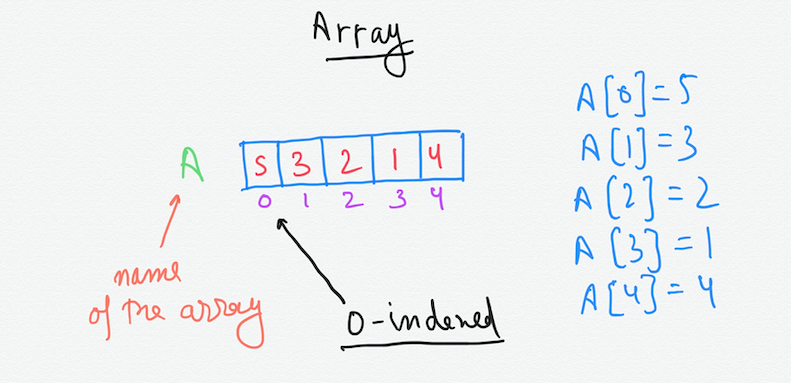In the above diagram, the name of the array is “A”. This array A has 5 elements({5,3,2,1,4}) that are stored sequentially and can be accessed by their index. The index of the first element is 0 so it is a zero-indexed array. The elements can be accessed by the ‘[]’ square bracket notation by giving the index of the element we want to access.

The pseudocode for Insertion Sort is-

``````INSERTION-SORT(A):

for j = 1 to A.length-1
key = A[j]
i = j-1
while i>=0 and A[i]>key
A[i+1] = A[i]
i=i-1
A[i+1] = key
``````

How can anyone believe that this algorithm does its job that is gives us the same set of numbers in ascending order always and halts.Donald Knuth is one of the pioneers in the computer sciences. You can read more about him on his wikipedia **page** .

Lets go through each iteration and see what operations the algorithm is performing underneath.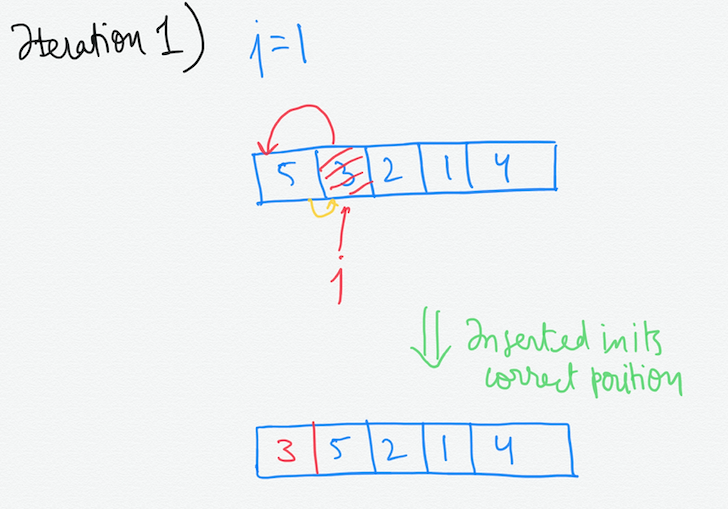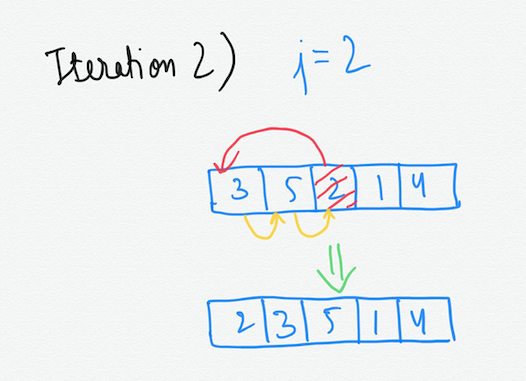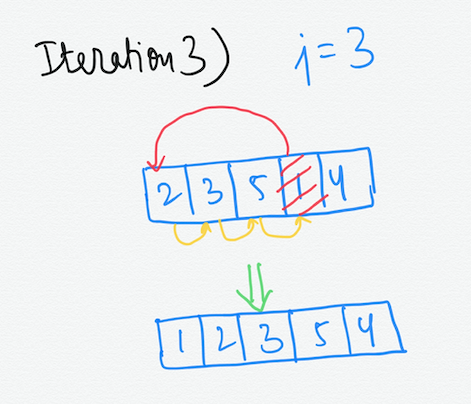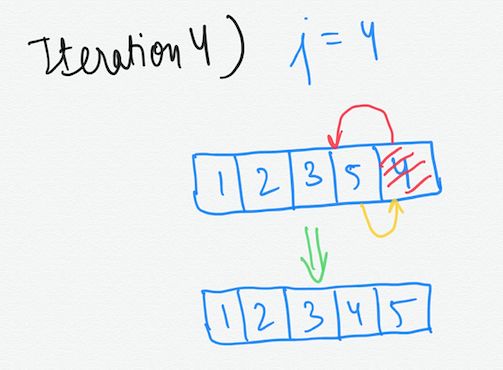Although it was not a detailed description, but you can see the movement of array elements on each iteration of the for loop. Also in pseudocode we use programming like constructs such as for, while, and, if, etc. Now this pseudocode can be easily implemented in any programming language of our choice.